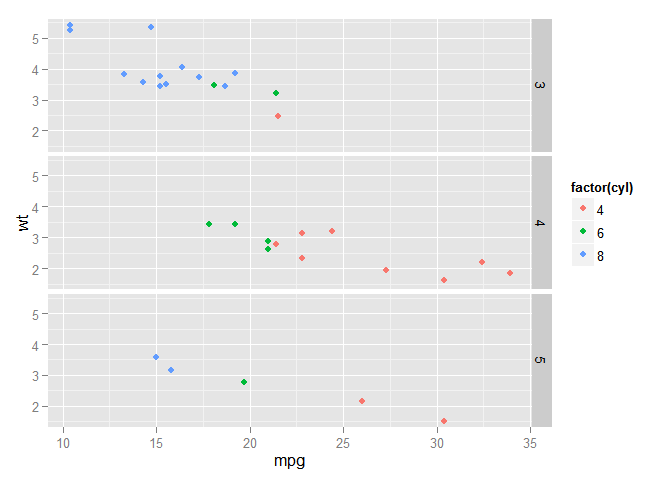facet: 另一种展示分组数据的方法

qplot(carat,price,color=color,data=diamonds,
geom='point') + geom_smooth(method='lm',size=1) +
scale_color_brewer(palette='Set1') +
facet_null()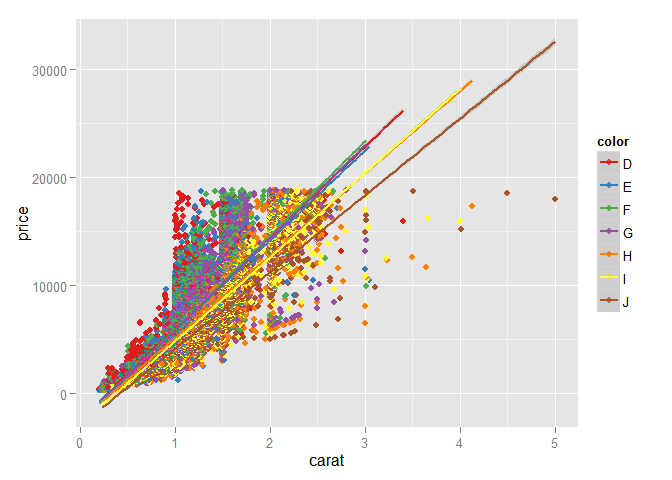facet_wrap(facets, nrow = NULL, ncol = NULL, scales = "fixed",
shrink = TRUE, as.table = TRUE, drop = TRUE)

**Arguments**

facets

nrow

ncol

scales

as.table

drop

p <- qplot(carat,price,color=color,data=diamonds,
geom='point') + geom_smooth(method='lm',size=1) +
scale_color_brewer(palette='Set1')
p + facet_wrap(~cut)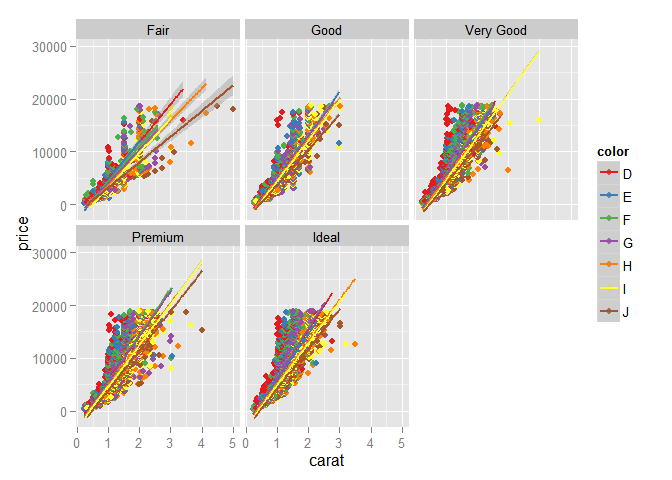p + facet_wrap(~cut, ncol=1)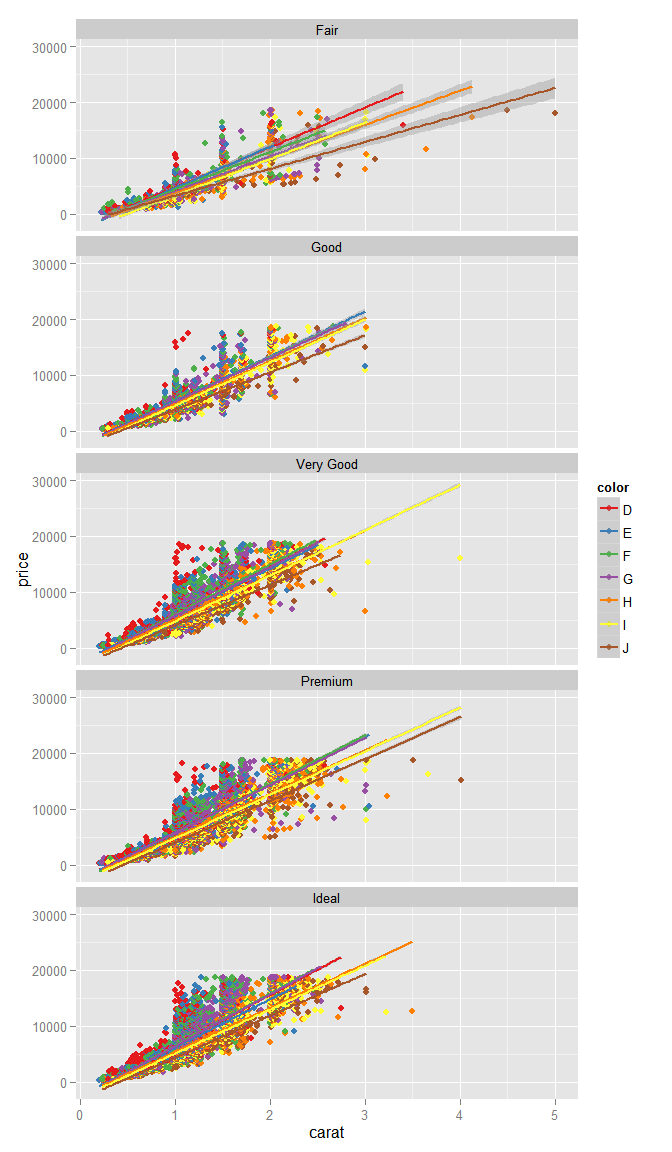p + facet_wrap(~cut+clarity, ncol=4)
## Warning in loop_apply(n, do.ply): NaNs produced
## Warning in loop_apply(n, do.ply): NaNs produced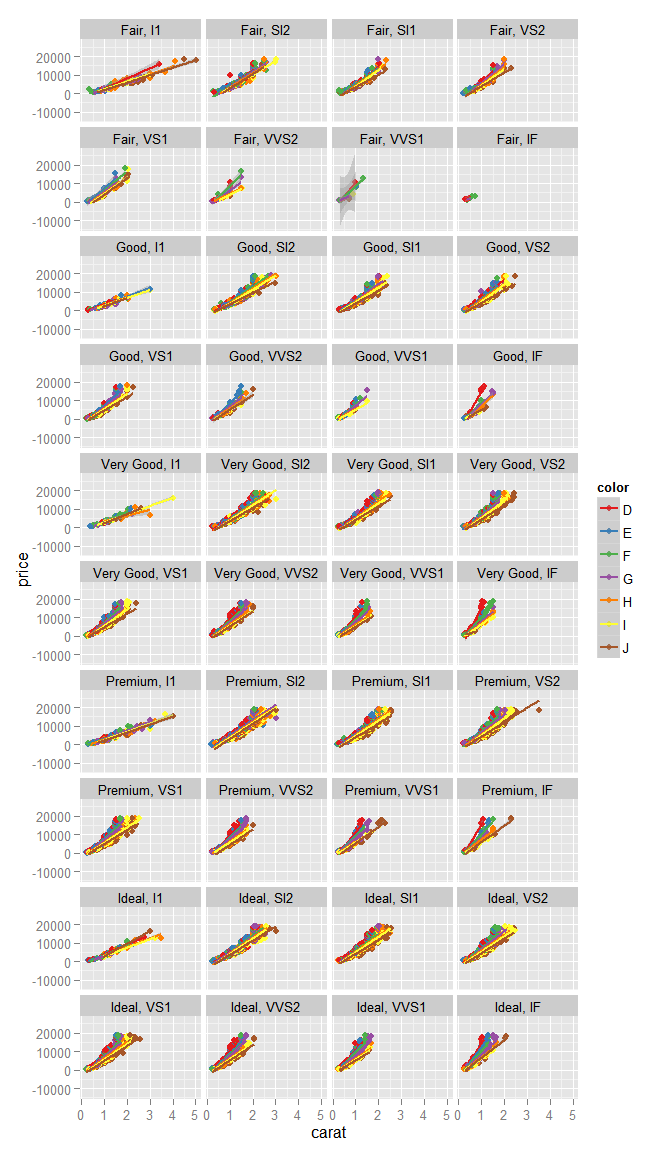p + facet_wrap(~clarity+cut, ncol=4, scales="free")
## Warning in loop_apply(n, do.ply): NaNs produced
## Warning in loop_apply(n, do.ply): NaNs produced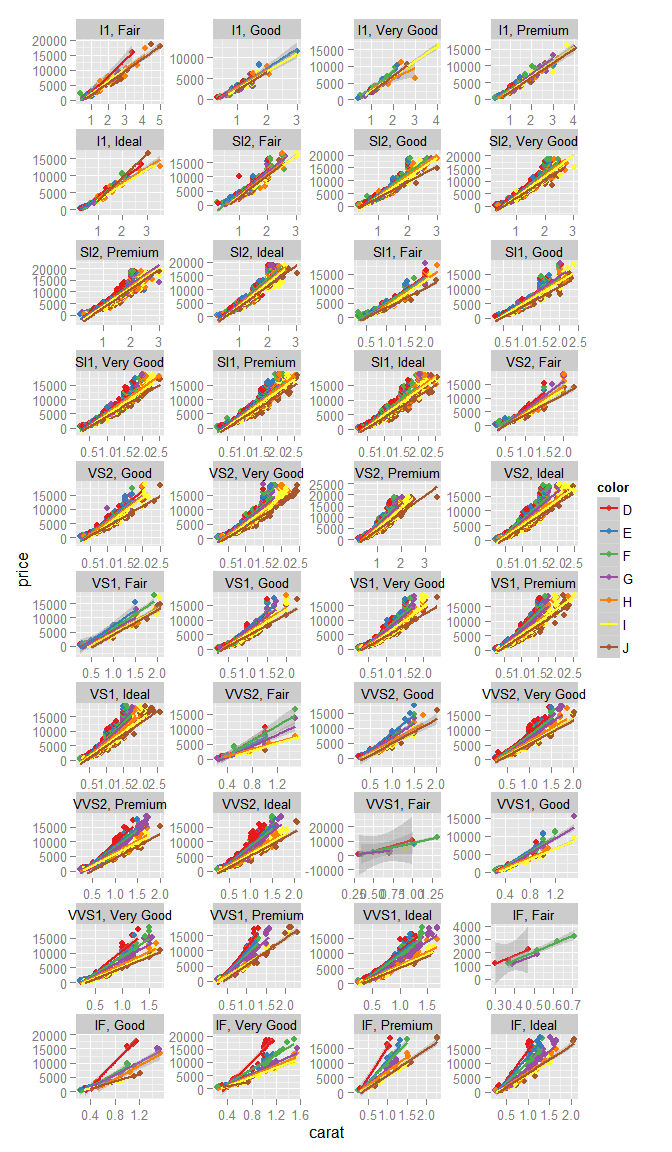p + facet_grid(clarity~cut)
## Warning in loop_apply(n, do.ply): NaNs produced
## Warning in loop_apply(n, do.ply): NaNs produced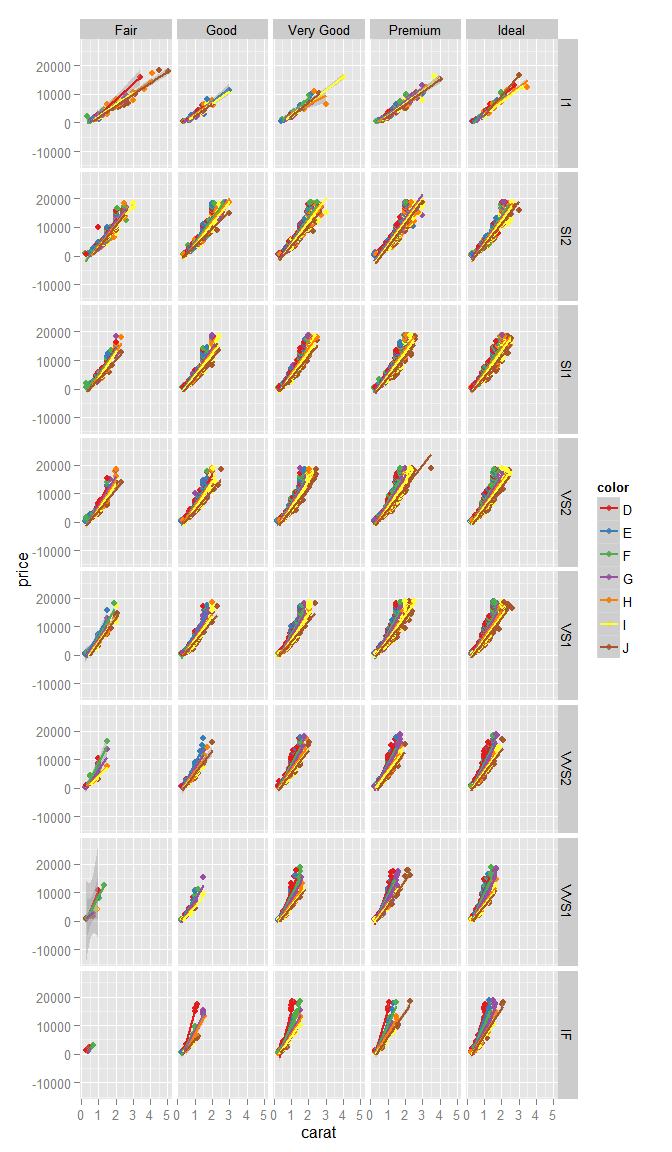facet_grid的选项中没有ncol和nrow，因为它们由数据的内容已经确定了，剩下的参数大部分和facet_wrap重合，其他参见文档。对于单行和单列的facet_wrapfacet_grid.~var, var~.是一致的(注意到grid的列变量的标签位置与wrap不同)。比如：

mt <- ggplot(mtcars, aes(mpg, wt, colour = factor(cyl))) + geom_point()

mt + facet_wrap(~gear, nrow=1)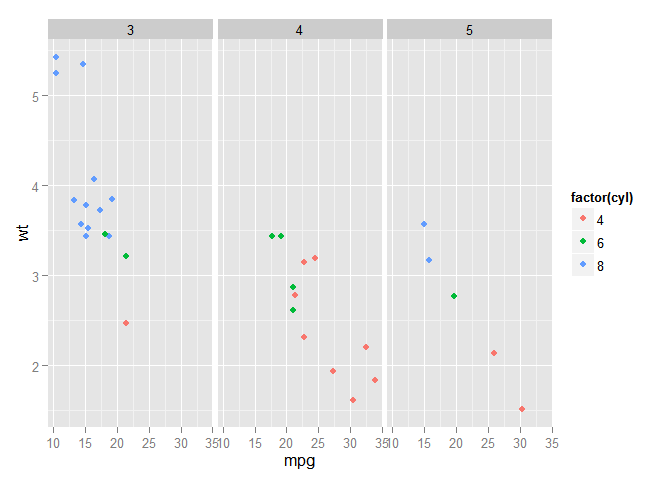mt + facet_grid(.~gear)mt + facet_wrap(~gear, ncol=1)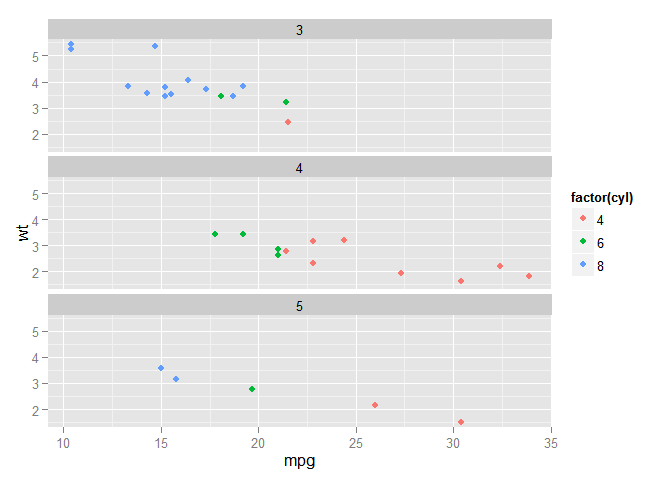mt + facet_grid(gear~.)